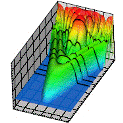## Research Papers in Physics and Astronomy## Anthony F. Starace Publications

November 1987

#### Comments

Published by American Institute of Physics and IEEE Computer Society; Computers in Physics 1, 70 (1987). Copyright 1987. Permission to use.

#### Abstract

Increasing interest in multiphoton absorption processes above the ionization threshold has led theorists to reexamine numerical techniques for calculating radiative transition matrix elements between states of a continuum electron moving at large radial distances in the field of an atom or an ion. Here it is shown that accurate free-free radial matrix elements may be obtained using the usual dipole length formula by means of a rotation at finite distance in the complex coordinate plane together with solution of the free-electron wave function's phase and amplitude at finite distance in the complex coordinate plane. The procedure is designed for use with numerically calculated wave functions for many electron atoms and ions. It avoids the use of analytic asymptotic formulas as well as transformation to the dipole acceleration formula and is accurate even for matrix elements between electron states that are close in energy, which is the case for which the alternative integration-by-parts method is inaccurate. We present comparisons of our numerical procedure with both analytic results and results of the integration-by-parts procedure for the case of free-free electron transitions in a pure Coulomb field.

COinS